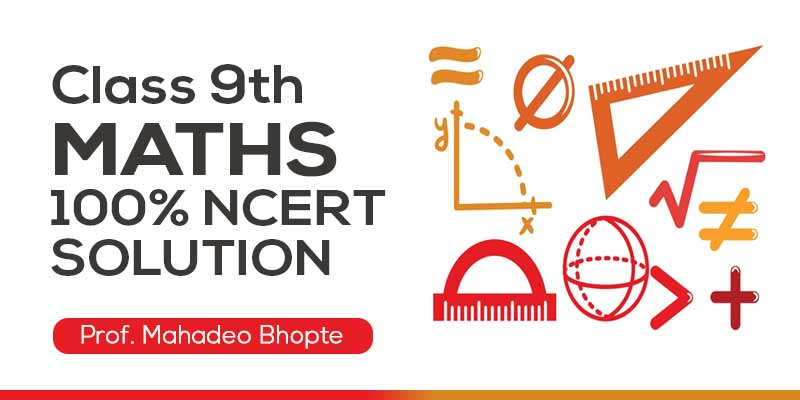## by Teach Tech Bhopal

4.7 (315 Users)
Class 9 , Maths
Board: CBSE & State Boards , Language: English

Mode- Online
Videos- 63
Validity- Till 31 Mar 2020
Language- English
No. of Views- Unlimited

## WHAT WILL I LEARN?

What will you get?

• उत्कृष्ट तथा विषय विशेषज्ञ द्वारा निर्मित
• कठिन सवालों का आसान तरीकों से हल
• 100% NCERT तथा अन्य बोर्ड के हल
• भूमिका / सूत्र व प्रमेय उत्पत्ति / प्रश्नावली हल / Tricks

## Curriculum

1. NUMBER SYSTEMS

2. POLYNOMIALS

• Basic/Exercise 2.1
• Basic/Exercise 2.2
• Basic/Exercise 2.3
• Basic/Exercise 2.4
• Basic/Exercise 2.5 (A)
• Basic/Exercise 2.5 (B)

3. COORDINATE GEOMETRY

• Basic
• Exercise 3.1, 3.2& 3.3

4. LINEAR EQUATIONS IN TWO VARIABLES

• Basic/Exercise 4.1
• Basic/Exercise 4.2
• Basic/Exercise 4.3
• Basic/Exercise 4.4

5. INTRODUCTION TO EUCLID’S GEOMETRY

• Basic/Exercise 5.1 & 5.2

6. LINES AND ANGLES

• Basic 6.1
• Exercise 6.1
• Basic/Exercise 6.2
• Basic/Exercise 6.3

7. TRIANGLES

• Basic/Exercise 7.1
• Basic/Exercise 7.2
• Basic/Exercise 7.3
• Basic/Exercise 7.4
• Basic/Exercise 7.5

• Basic 8.1
• Basic/Exercise 8.1
• Basic/Exercise 8.2

9. AREAS OF IIgms AND TRIANGLES

• Basic/Exercise 9.1 Demo
• Basic/Exercise 9.2
• Basic/Exercise 9.3 (A)
• Basic/Exercise 9.3 (B)
• Basic/Exercise 9.4 (A)
• Basic/Exercise 9.4 (B)

10. CIRCLES

• Basic/Exercise 10.1
• Basic/Exercise 10.2
• Basic/Exercise 10.3
• Basic/Exercise 10.4
• Basic/Exercise 10.5
• Basic/Exercise 10.6

11. CONSTRUCTIONS

• Basic/Exercise 11.1
• Basic/Exercise 11.2

12. HERON’S FORMULA

• Basic/Exercise 12.1
• Basic/Exercise 12.2

13. SURFACE AREAS AND VOLUMES

• Basic/Exercise 13.1
• Basic/Exercise 13.2
• Basic/Exercise 13.3
• Basic/Exercise 13.4
• Basic/Exercise 13.5
• Basic/Exercise 13.6
• Basic/Exercise 13.7
• Basic/Exercise 13.8
• Basic/Exercise 13.9
• Formula Summary 13.10

14. STATISTICS

• Basic/Exercise 14.1
• Basic/Exercise 14.2
• Basic/Exercise 14.3
• Basic/Exercise 14.4

15. PROBABILITY

• Basic/Exercise 15.1
• Basic/Exercise 15.2

## About Teach Tech Bhopal### Teach Tech Bhopal

The objective of the “Teach Tech” is to teach students who are deprived of high-level education in rural area. They provide recorded lectures in very simple language in the form of DVD, so the students can study, sitting at home with their convenient time through DVD player or computer. Many students have achieved good positions in the examination by studying our Teach Tech DVDs.

## 3500 2300

Includes 18 % GST

Mode- Online

Videos- 63

Validity- Till 31 Mar 2020

Language- English

No. of Views- Unlimited